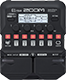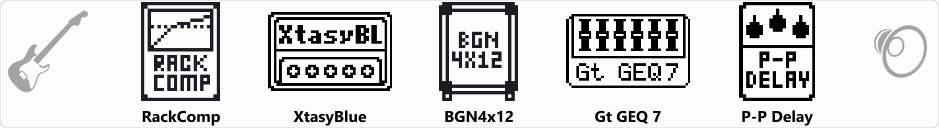# Timmons Low

Discussion in 'Zoom G1/G1X Four' started by Andreson, Feb 17, 2021.

1. Timmons LowDevice: Zoom G1 Four
Firmware: 2.00

Name on device: Timmons Lo
Optimized for: Phones/Speaker

Effects chain:Effect: "RackComp" (Dynamics), active - "yes"
"Threshold" = 40
"Ratio" = 6
"Attack" = 7
"Volume" = 80

Effect: "XtasyBlue" (Amp simulator), active - "yes"
"Bass" = 50
"Middle" = 50
"Treble" = 85
"Presence" = 40
"STRCT" = LO
"Gain" = 30
"Volume" = 20

Effect: "BGN4x12" (Cabinet), active - "yes"
"MIC" = ON
"D57: D421" = 50
"Hi" = 75
"Lo" = 60

Effect: "Gt GEQ 7" (Filter), active - "yes"
"100" = 0.0
"200" = 0.0
"400" = 0.0
"800" = 0.0
"1.6k" = 1.5
"3.2k" = 2.5
"6.4k" = -2.0
"VOL" = 80

Effect: "P-P Delay" (Delay), active - "yes"
"Time" = 700
"F.B." = 30
"Mix" = 40
"Tail" = On

Patch Volume: 100